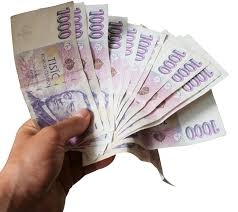# Two-thirds 7319

Bohuš spent a third of his savings on groceries. He paid two-thirds of the amount he had left at the stationery store. After shopping, he still had €18 left. How much € did Bohuš initially have?

x =  81 Eur

### Step-by-step explanation:

x/3 + 2/3 · (x-x/3) + 18 = x

0.666667x = 54

x = 54/0.66666667 = 81

x = 81

Our simple equation calculator calculates it.Did you find an error or inaccuracy? Feel free to write us. Thank you!

Tips for related online calculators
Need help calculating sum, simplifying, or multiplying fractions? Try our fraction calculator.
Do you have a linear equation or system of equations and looking for its solution? Or do you have a quadratic equation?